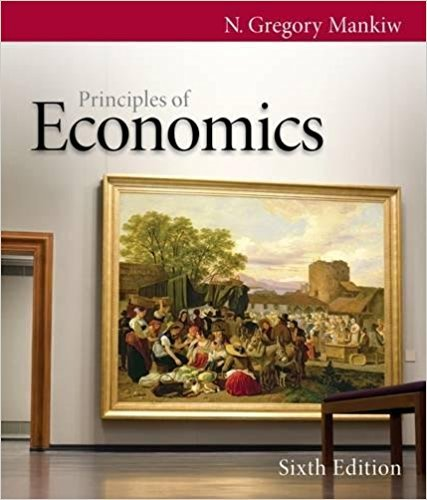×
Get Full Access to Principles Of Economics - 6 Edition - Chapter 5 - Problem Problems and applications 5.5
Get Full Access to Principles Of Economics - 6 Edition - Chapter 5 - Problem Problems and applications 5.5

×

Textbooks / Business / Principles of Economics 6 / Chapter 5 / Problem Problems and Applications 5.5

# The equilibrium price of coffee mugs rose sharply last month, but the equilibriumISBN: 9780538453059 472

## Solution for problem Problems and Applications 5.5 Chapter 5

Principles of Economics | 6th Edition

• Textbook Solutions
• 2901 Step-by-step solutions solved by professors and subject experts
• Get 24/7 help from StudySoup virtual teaching assistantsPrinciples of Economics | 6th Edition

4 5 1 393 Reviews
27
3
Problem Problems and Applications 5.5

The equilibrium price of coffee mugs rose sharply last month, but the equilibrium quantity was the same as ever. Three people tried to explain the situation. Which explanations could be right? Explain your logic. Billy: Demand increased, but supply was totally inelastic. Marian: Supply increased, but so did demand. Valerie: Supply decreased, but demand was totally inelastic.

Step-by-Step Solution:
Step 1 of 3

SCM 200 week 7 notes E(statistic)= parameter E(x bar)= mu Point and interval estimates: • a point estimate is a single # that is used to estimate an unknown population parameter an interval estimate (or confidence interval) is a range of #’s thought to contain • an unknown parameter value T/F All standard deviations are standard errors, but not all standard errors are standard deviations.........F T/F A sampling distribution is a distribution of all possible values of a statistic for a give sample size.....T T/F Given a population standard deviation, as a sample size increases, standard error also increases....F

Step 2 of 3

Step 3 of 3

##### ISBN: 9780538453059

Principles of Economics was written by and is associated to the ISBN: 9780538453059. This textbook survival guide was created for the textbook: Principles of Economics, edition: 6. The full step-by-step solution to problem: Problems and Applications 5.5 from chapter: 5 was answered by , our top Business solution expert on 03/16/18, 04:26PM. This full solution covers the following key subjects: . This expansive textbook survival guide covers 36 chapters, and 670 solutions. Since the solution to Problems and Applications 5.5 from 5 chapter was answered, more than 1411 students have viewed the full step-by-step answer. The answer to “The equilibrium price of coffee mugs rose sharply last month, but the equilibrium quantity was the same as ever. Three people tried to explain the situation. Which explanations could be right? Explain your logic. Billy: Demand increased, but supply was totally inelastic. Marian: Supply increased, but so did demand. Valerie: Supply decreased, but demand was totally inelastic.” is broken down into a number of easy to follow steps, and 57 words.

Unlock Textbook Solution